@77qingliu 2018-05-21T00:21:50.000000Z 字数 3860 阅读 1064

# 拒绝演绎

信用评分

## 定义和理由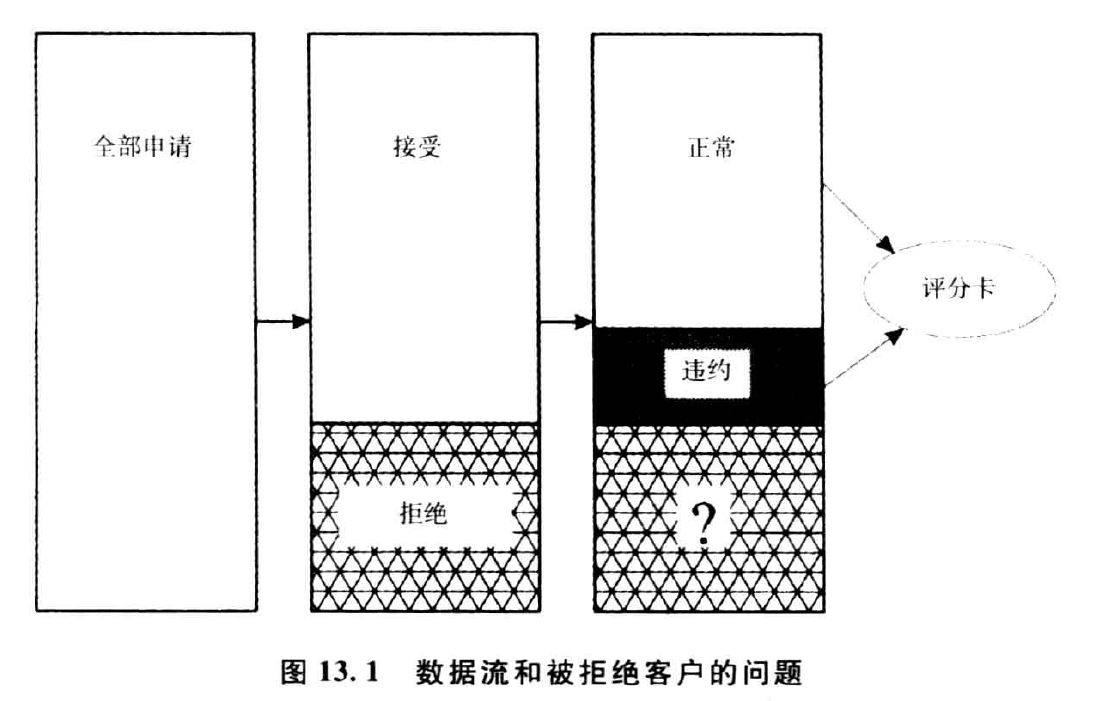## 拒绝演绎的方法

1. 简单赋值方法。由分析人员将被拒绝的账户指定为两种账户状态中的一种，即正常或违约。
2. 通过外推法将模型范围扩展到被拒绝记录的方法，称为强化法。
演绎被拒绝申请的真实状态的唯一方法是系统性地接受拒绝申请记录中一个样本并监测真实表现随时间的变化，度量他们的违约行为。然后，假设其余被拒绝申请具有相似的违约分布。尽管这是最可靠的方法，但由于放任某些账户发生违约的相关成本而使其并不具有可行性。

## 简单赋值法

### 比例赋值

%PropAssign根据随机选择实施状态值的比例赋值，其参数如下：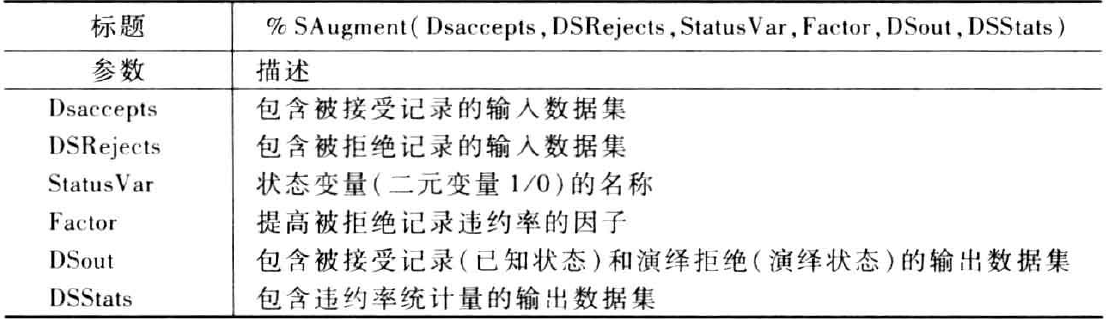data acc; do i=1 to 1000;  score = int(ranuni(0)*1000);  status=0;if score <200 and ranuni(0)>0.8 then status=1; if score>=200 and score<400 and ranuni(0)>0.85 then status=1; if score>=400 and score<600 and ranuni(0)>0.90 then status=1; if score>=600 and score<800 and ranuni(0)>0.95 then status=1; if score>=800 and ranuni(0)>0.98 then status=1;    output ;end;run;data rej; do i=1 to 1000;  score = int(ranuni(0)*1000);   output ;end;run;/*******************************************************//* Call the macro  *//*******************************************************/%let DSAccepts=acc;%let DSRejects=rej;%let statusVar=status;%let Factor =1.5;%let DSout=AllData;%let DSStats=stats;%PropAssign(&DsAccepts, &DSRejects, &StatusVar, &Factor, &DSout, &DSStats);proc print data=stats;run;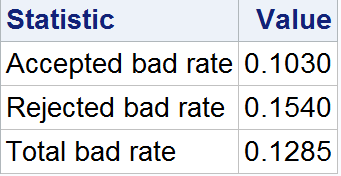## 强化法

### 简单强化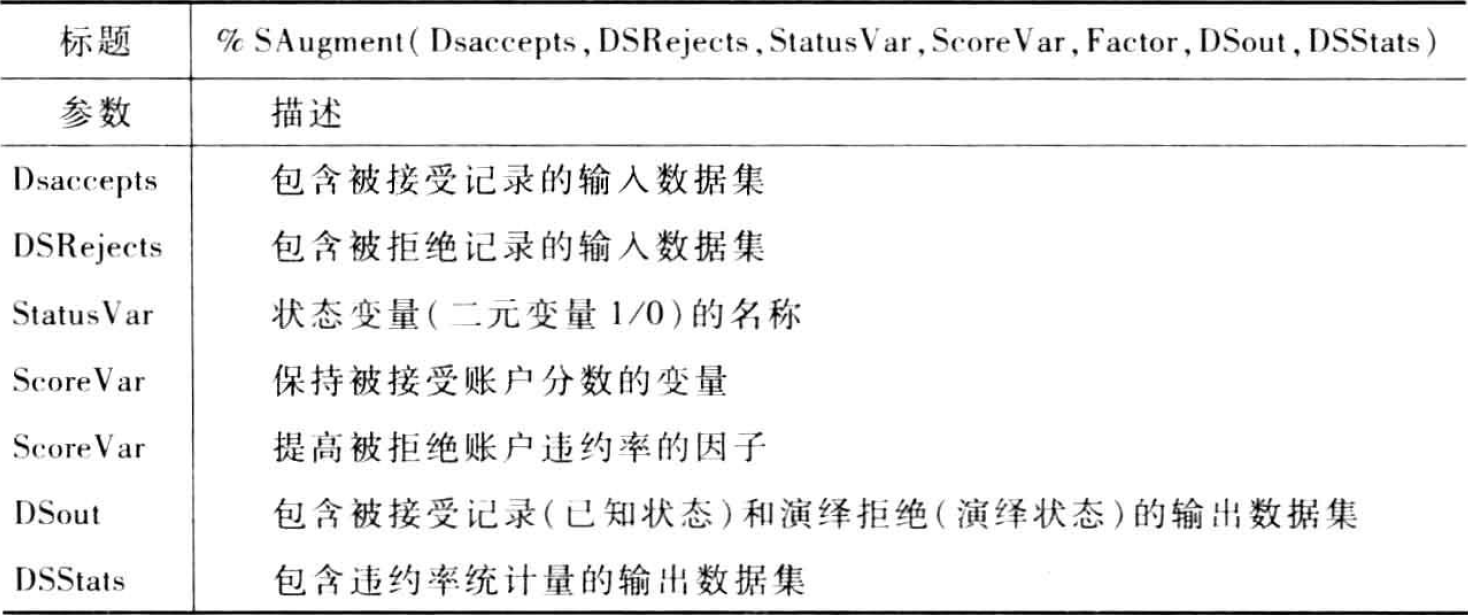data acc; do i=1 to 1000;  score = int(ranuni(0)*1000);  status=0;if score <200 and ranuni(0)>0.8 then status=1; if score>=200 and score<400 and ranuni(0)>0.85 then status=1; if score>=400 and score<600 and ranuni(0)>0.90 then status=1; if score>=600 and score<800 and ranuni(0)>0.95 then status=1; if score>=800 and ranuni(0)>0.98 then status=1;    output ;end;run;data rej; do i=1 to 1000;  score = int(ranuni(0)*1000);   output ;end;run;/* Call the macro  */%SAugment(acc, rej, status, Score, 5, all, stats);proc print data=stats;run;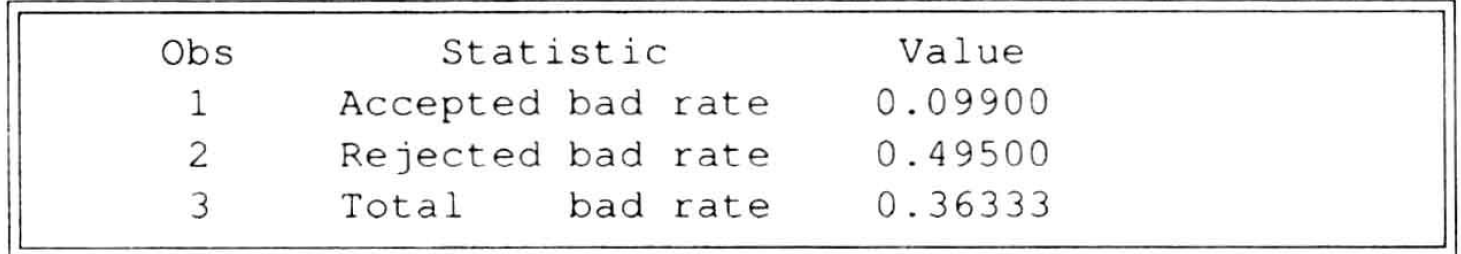### 模糊强化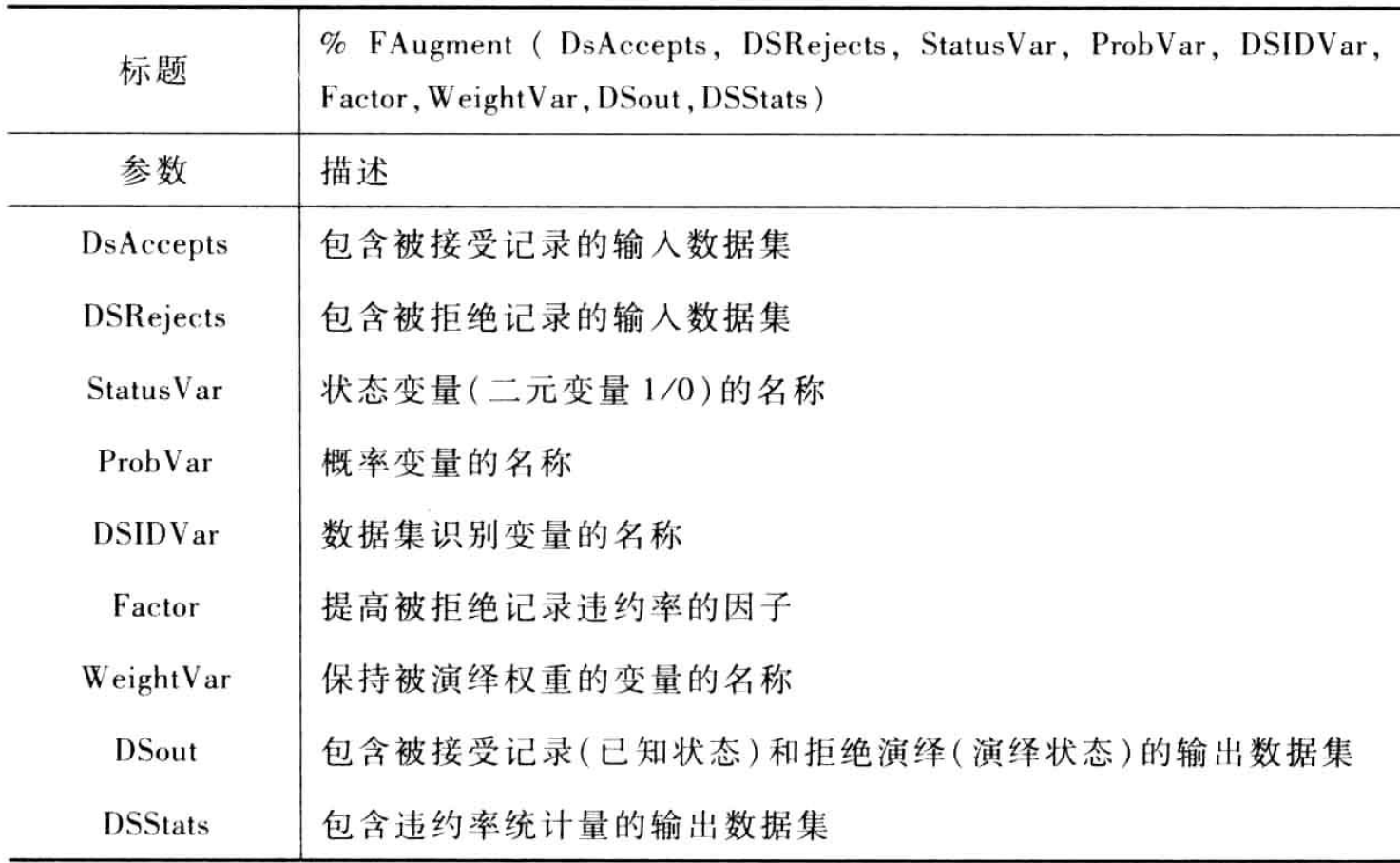data acc; do i=1 to 1000;  Prob = 1-ranuni(0);  status=0;  if Prob <0.2 and  ranuni(0)>0.8 then status=1;   if prob>=0.2 and prob<0.4 and  ranuni(0)>0.85 then status=1;   if Prob>=.4 and prob<0.6 and ranuni(0)>0.90 then status=1;   if Prob>=0.6 and prob<0.8 and ranuni(0)>0.95 then status=1;   if prob>=0.8 and ranuni(0)>0.98 then status=1;    output ;end;run;data rej; do i=1 to 1000;  Prob= ranuni(0);   output ;end;run;/* Call the macro  */%FAugment(acc, rej, Status, Prob, _source, 5, Weight ,all, Stats);proc print data=stats;run;

### 打包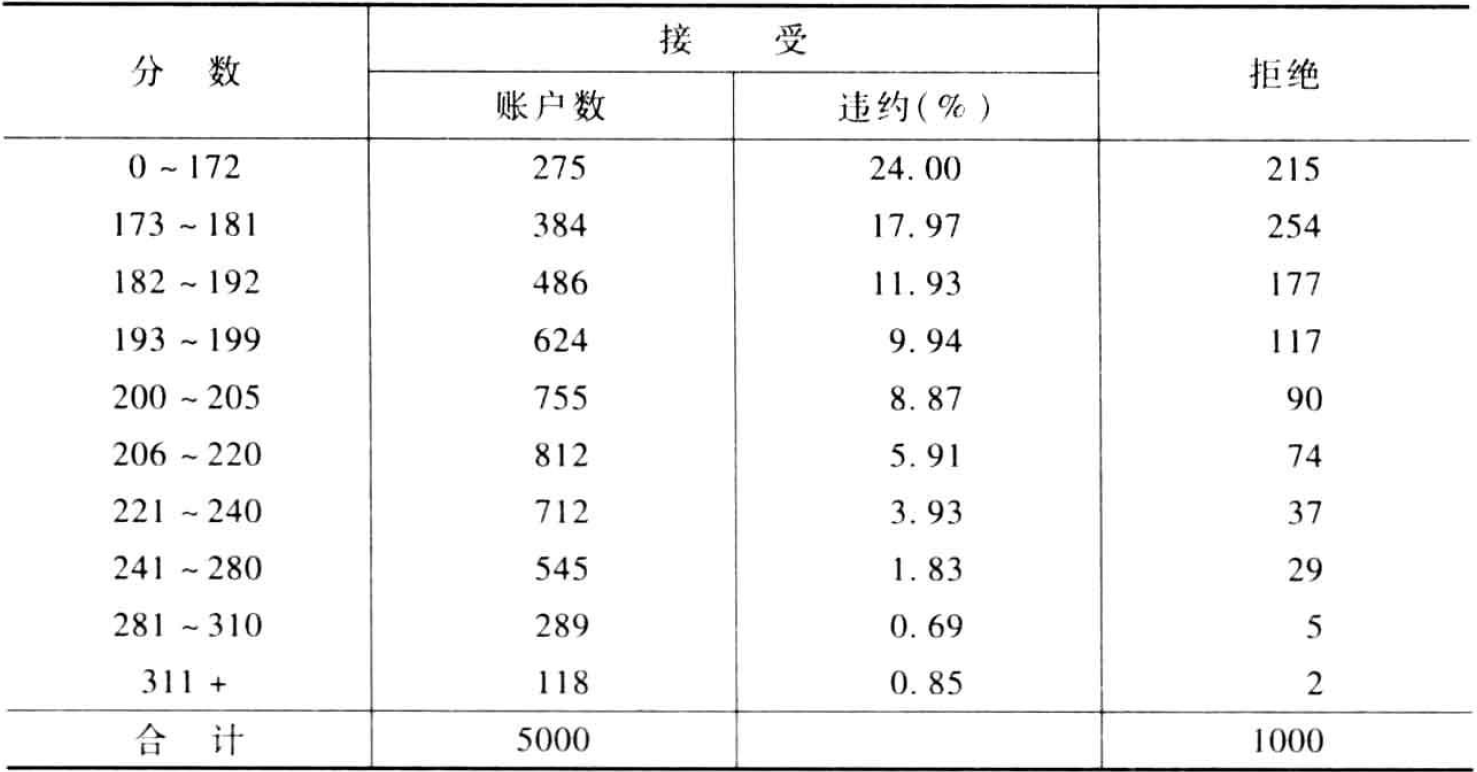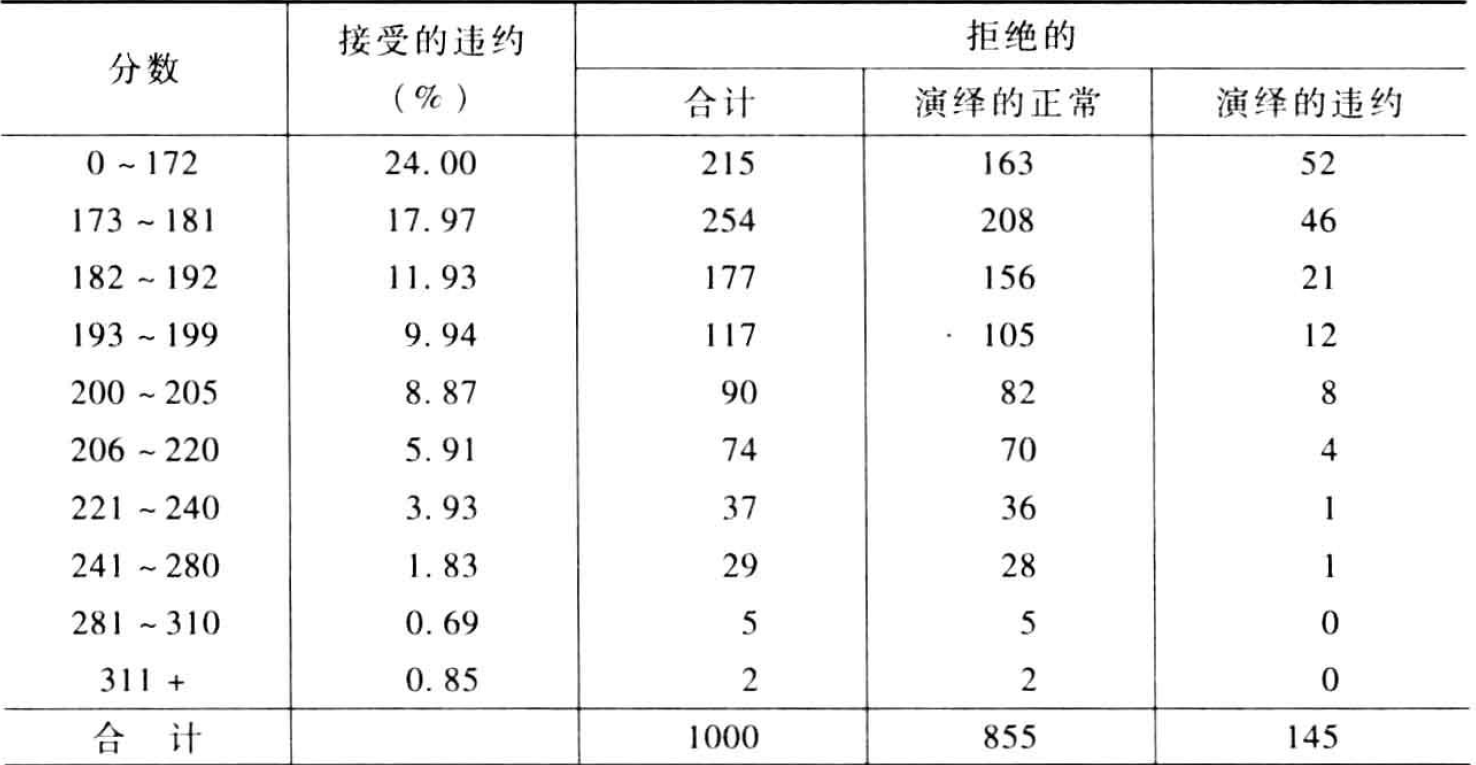%Parceling用一个简单的因子实施打包强化法，以提高拒绝记录的坏账率，其参数如下：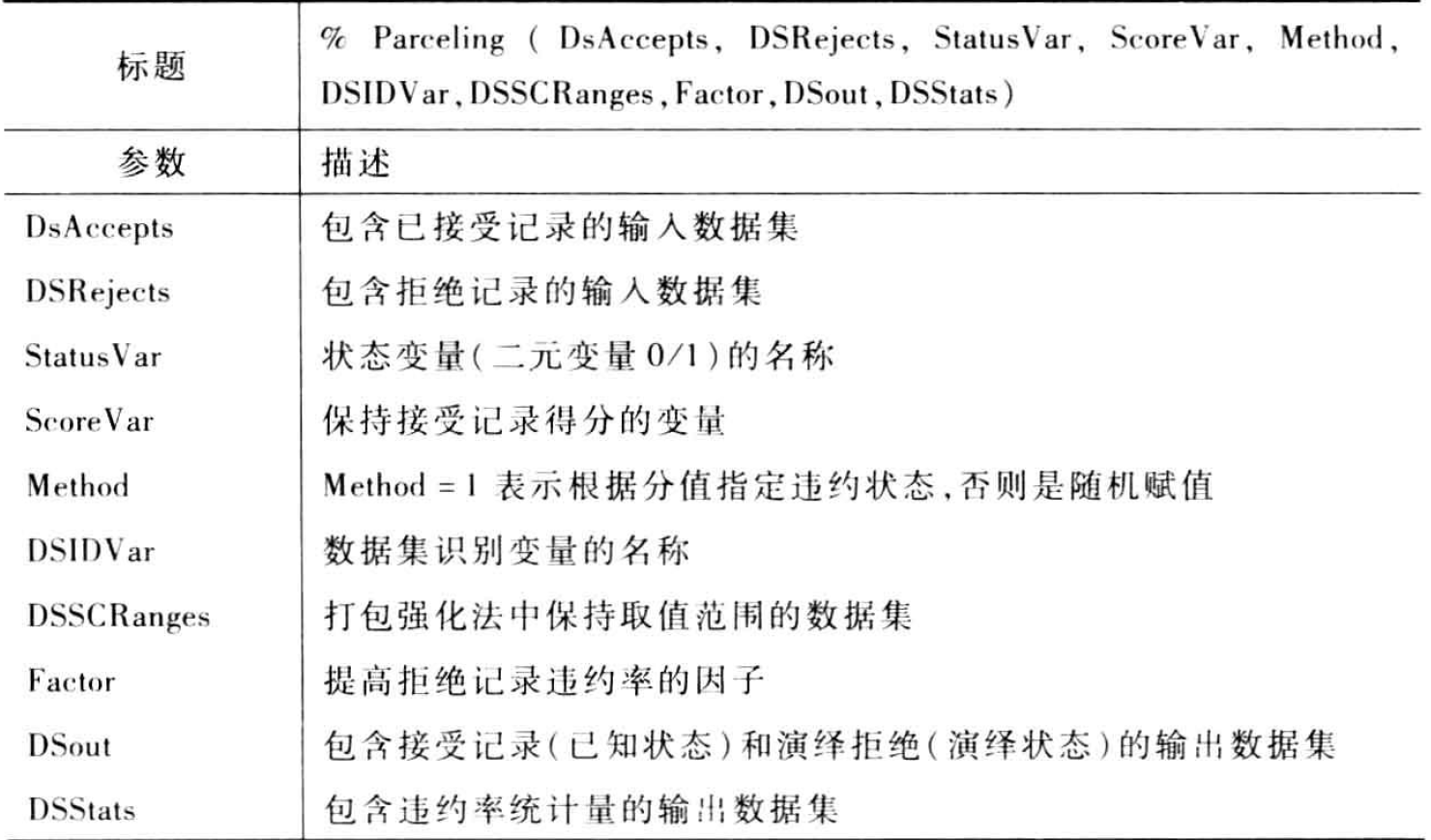data acc; do ID=1 to 1000;  score = int(ranuni(0)*1000);  status=0;if score <200 and ranuni(0)>0.8 then status=1; if score>=200 and score<400 and ranuni(0)>0.85 then status=1; if score>=400 and score<600 and ranuni(0)>0.90 then status=1; if score>=600 and score<800 and ranuni(0)>0.95 then status=1; if score>=800 and ranuni(0)>0.98 then status=1;    output ;end;run;data ranges;input LowerLimit UpperLimit;datalines;0    100100  200200 300300 400400 500500 600600 700700 800800 900900 1000;run;data rej; do ID=1001 to 2000;  score = int(ranuni(0)*1000);   output ;end;run;/* Call the macro  */%Parceling(acc, rej, Status, Score, 2, _source, Ranges, 2, all, Stats);proc print data=stats;run;

## 拒绝演绎的应用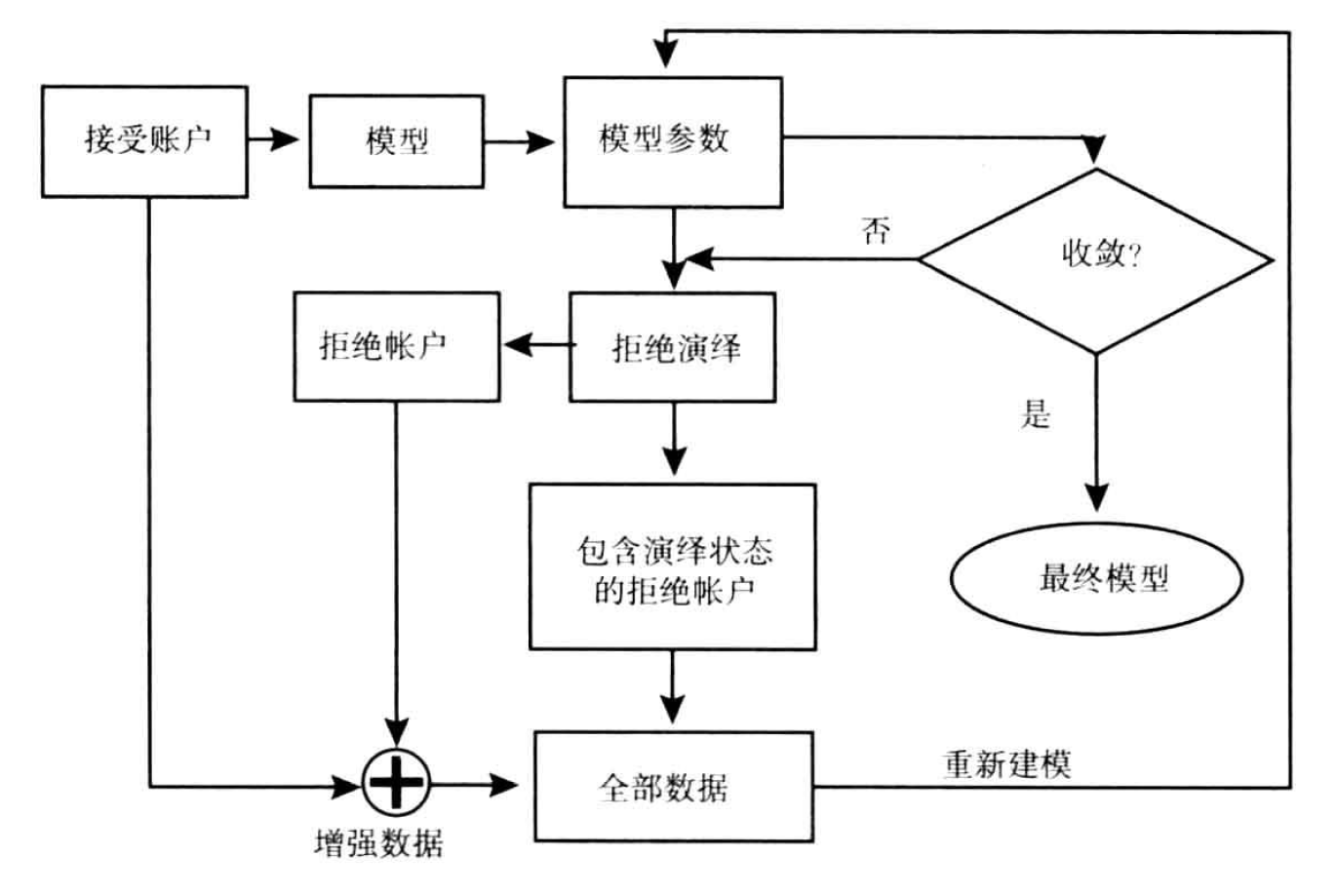• 模型参数值的收敛
• 接收和拒绝记录中违约的概率估计的收敛
• 经过几次迭代过程后，分值的频率分布在某些预先设定的分值区间变得稳定。• 私有
• 公开
• 删除